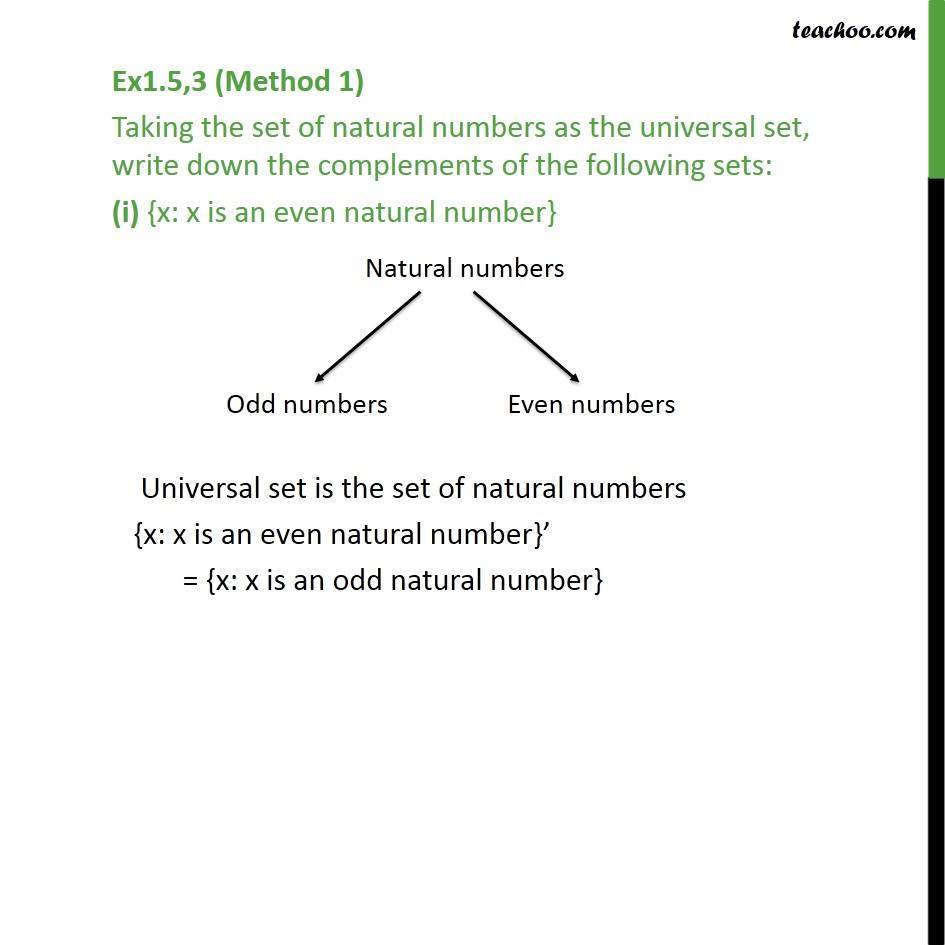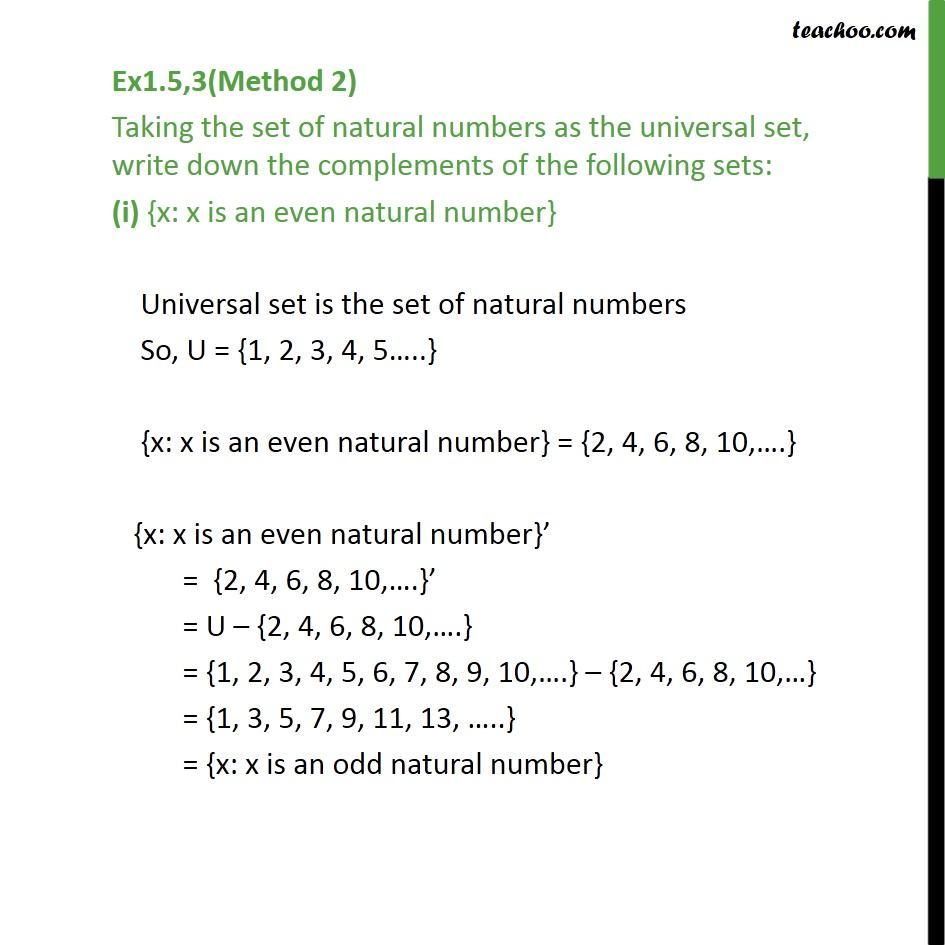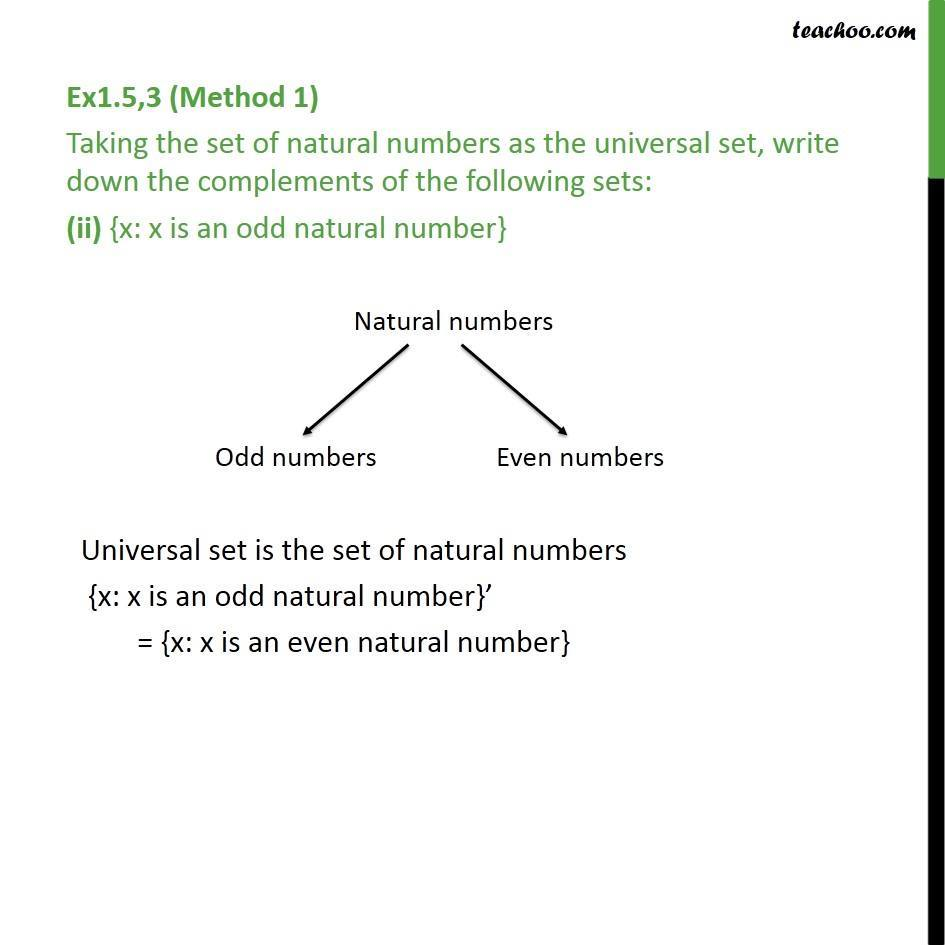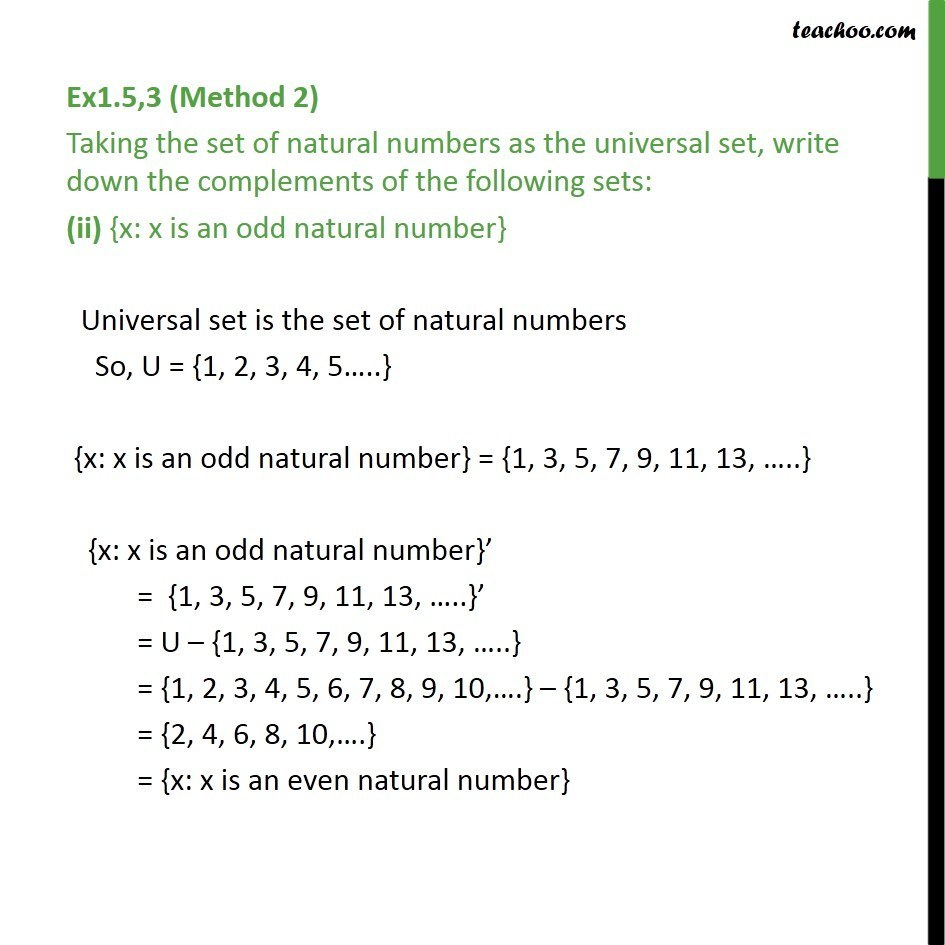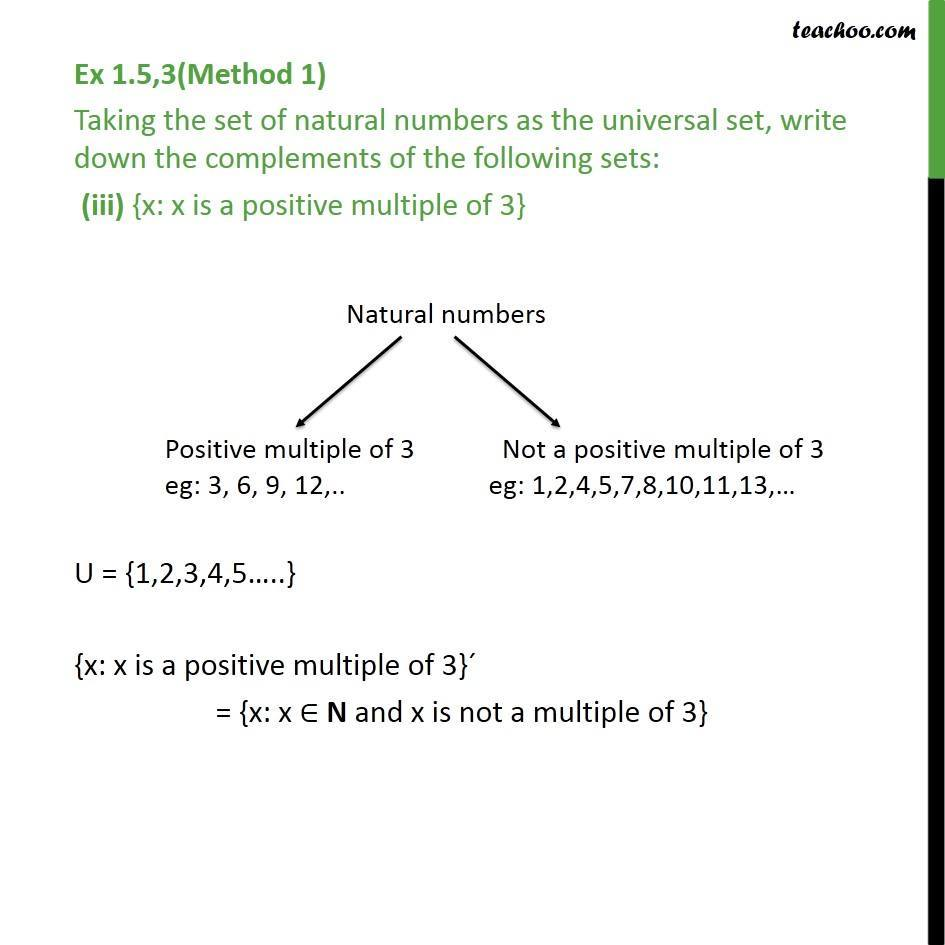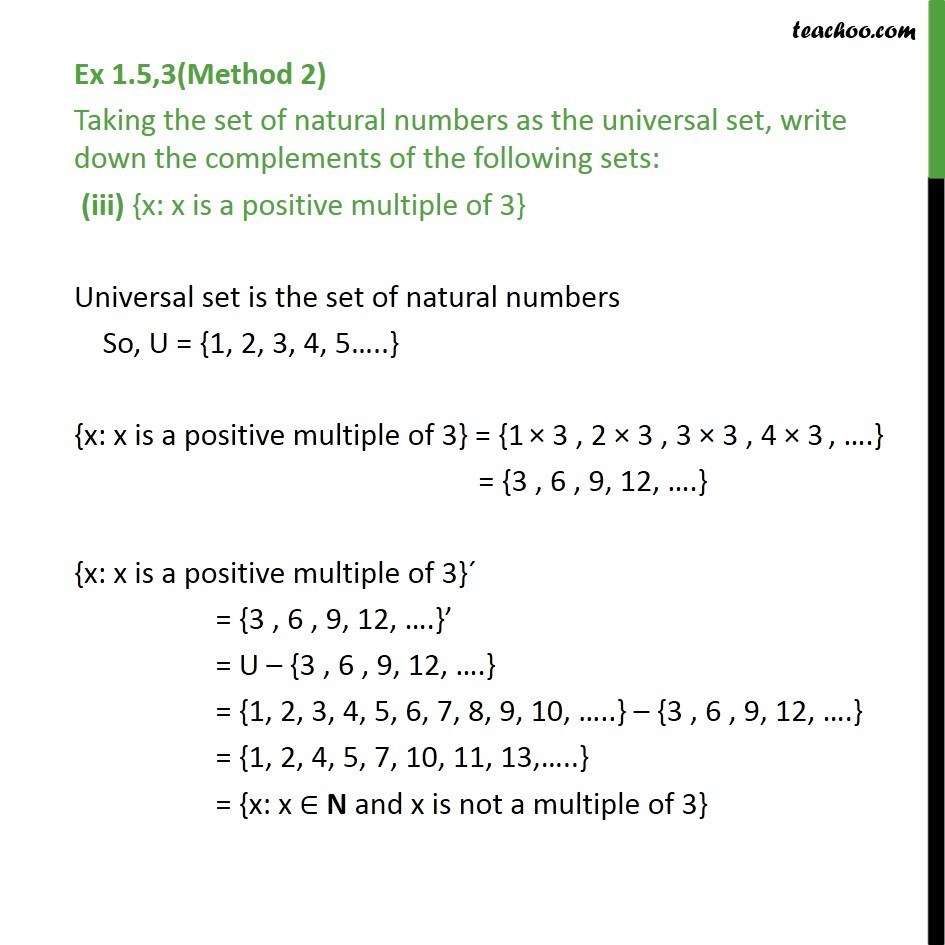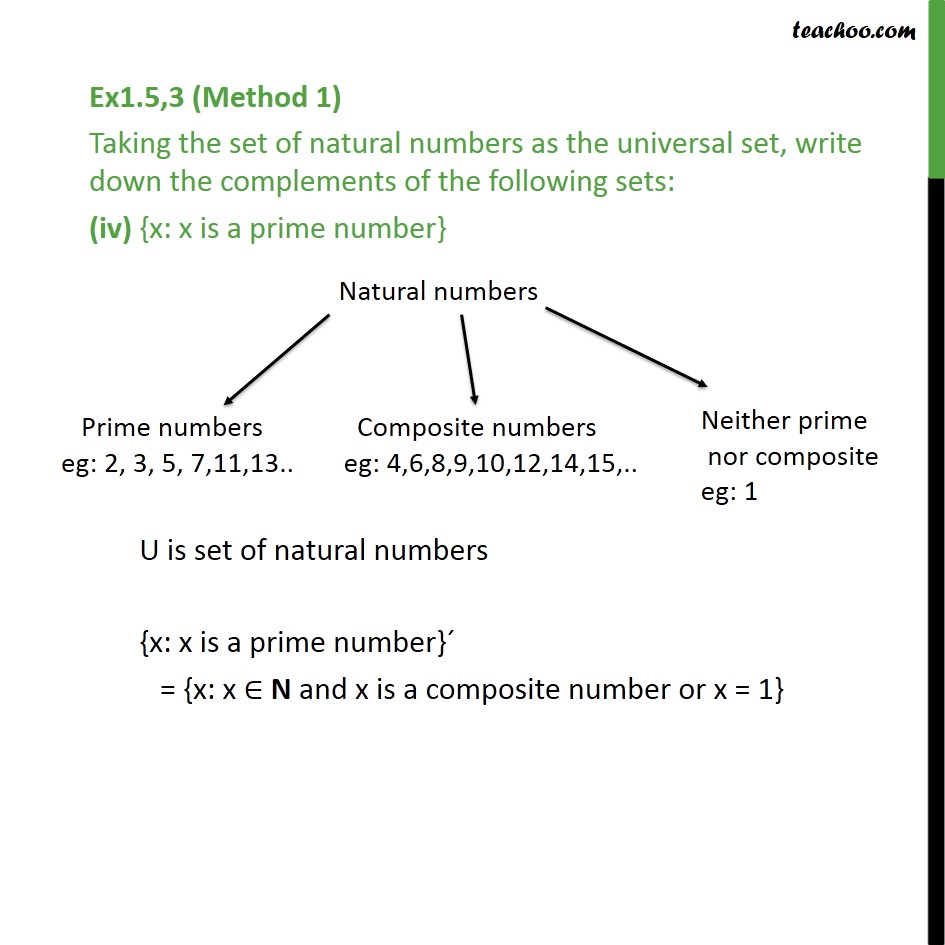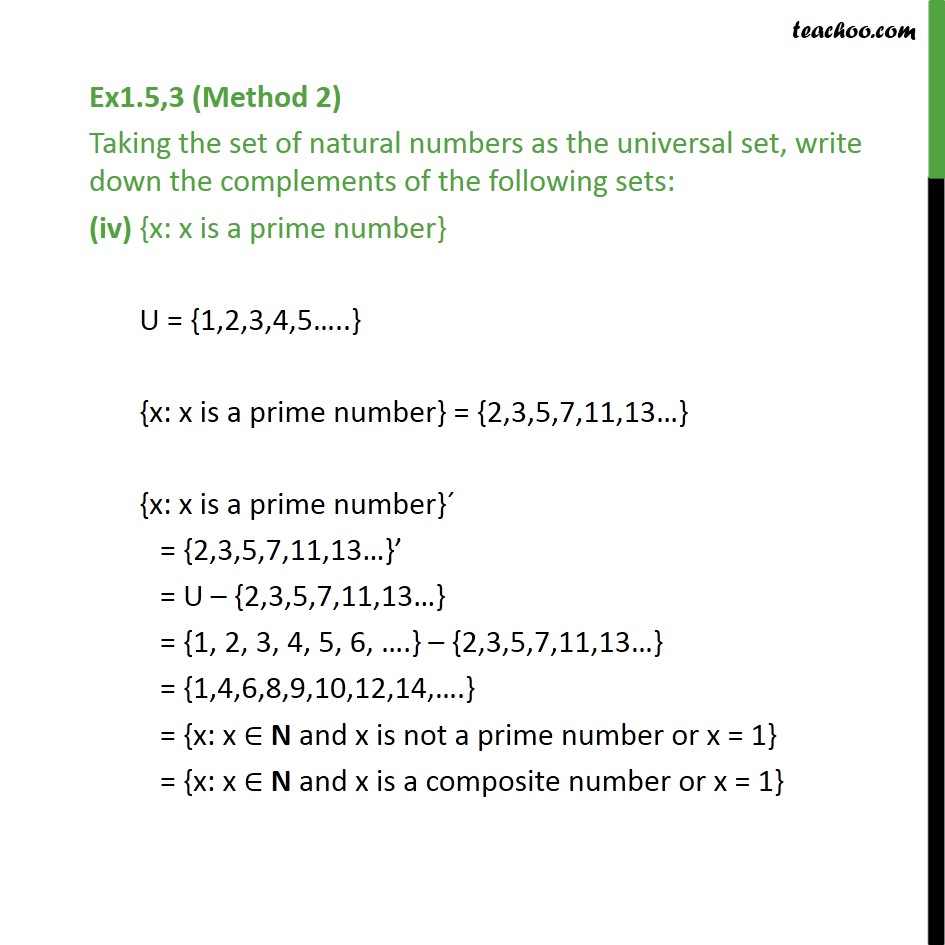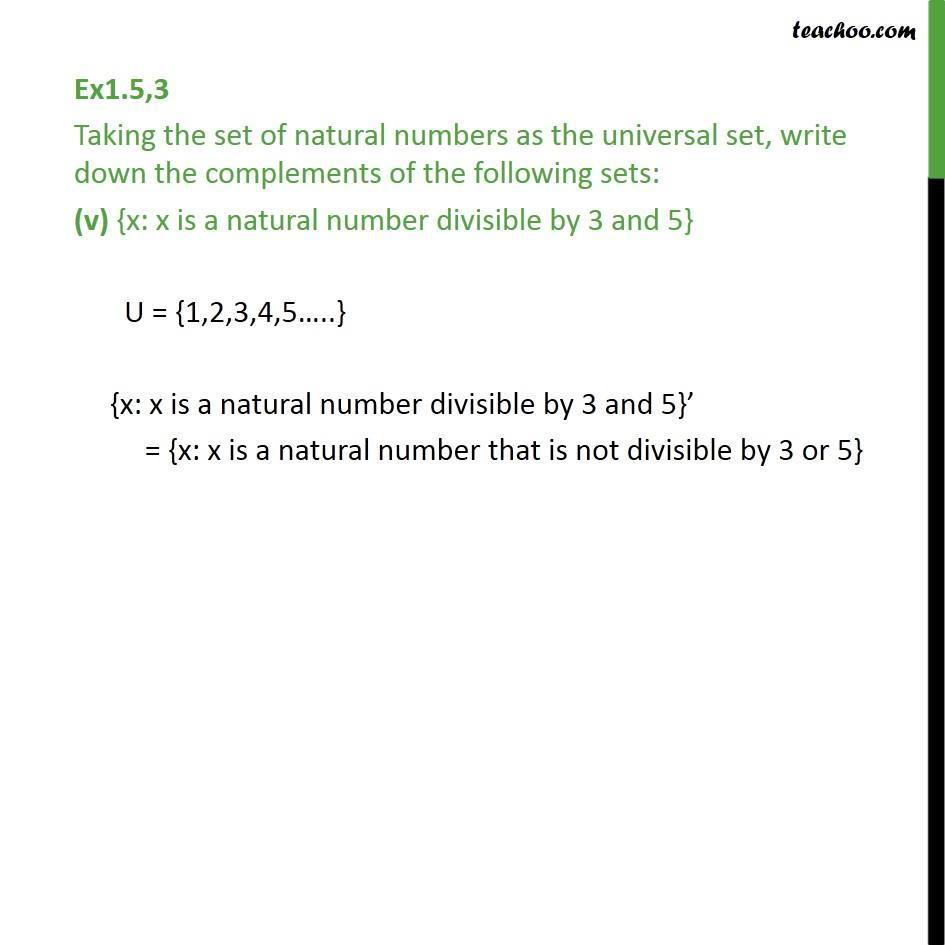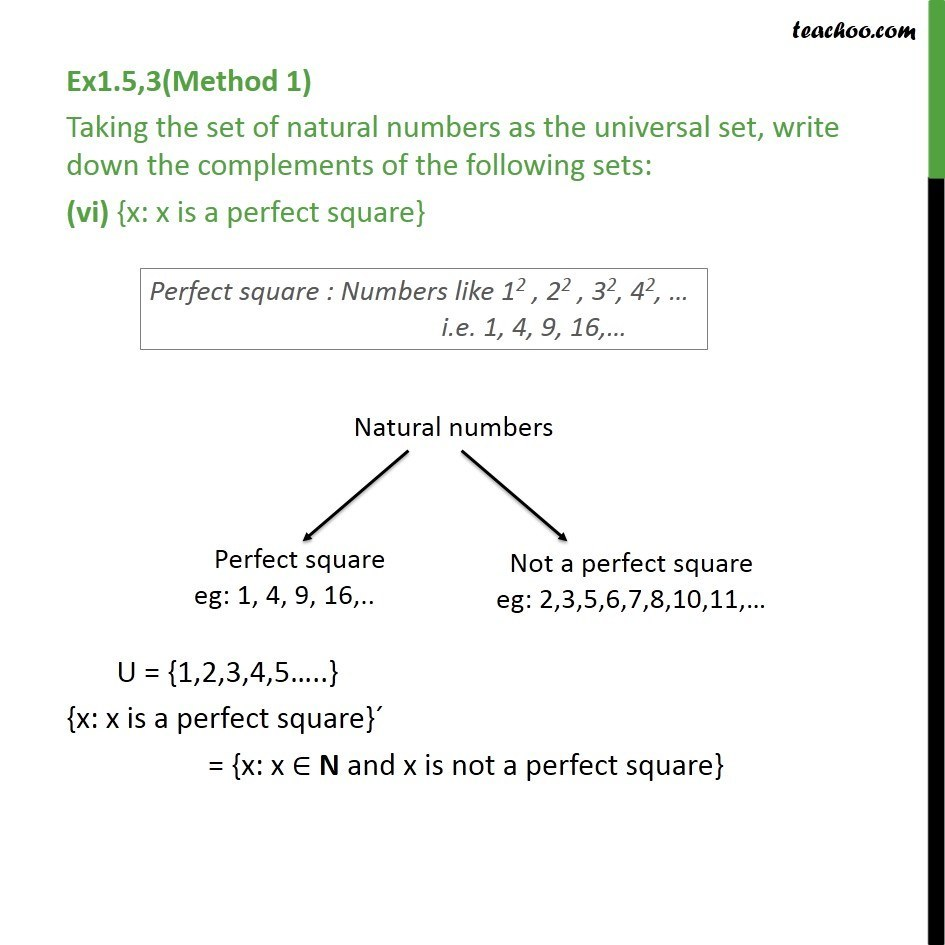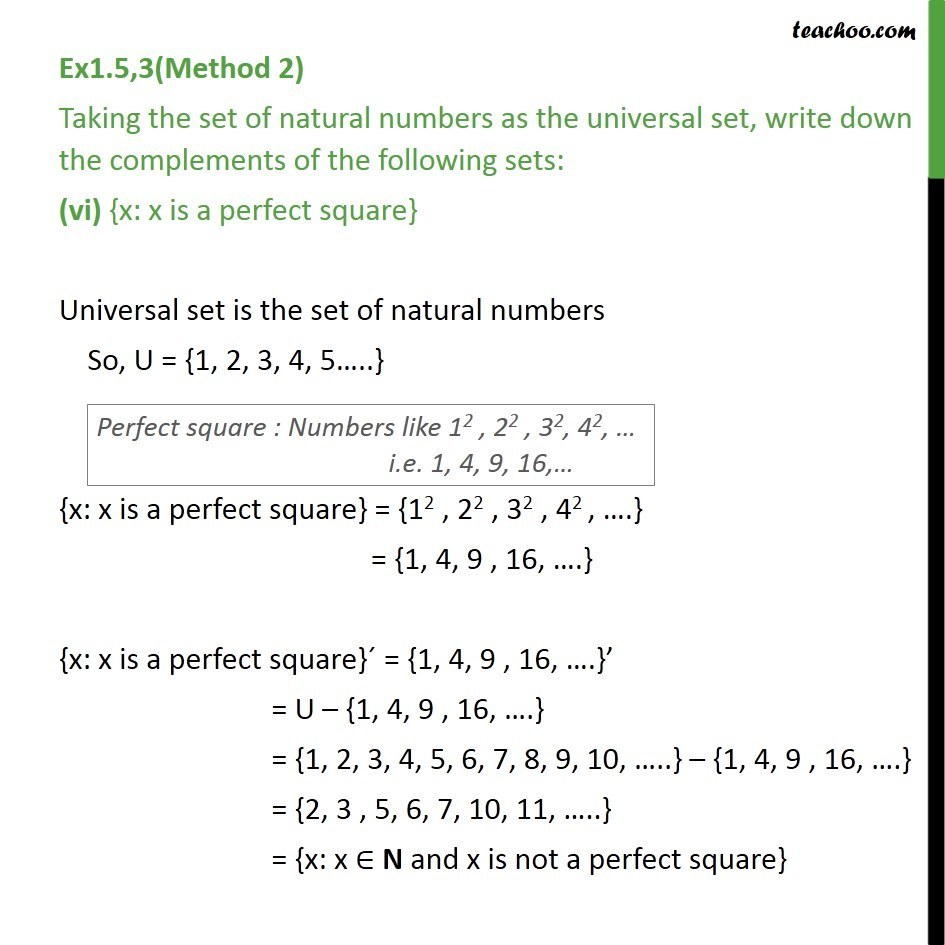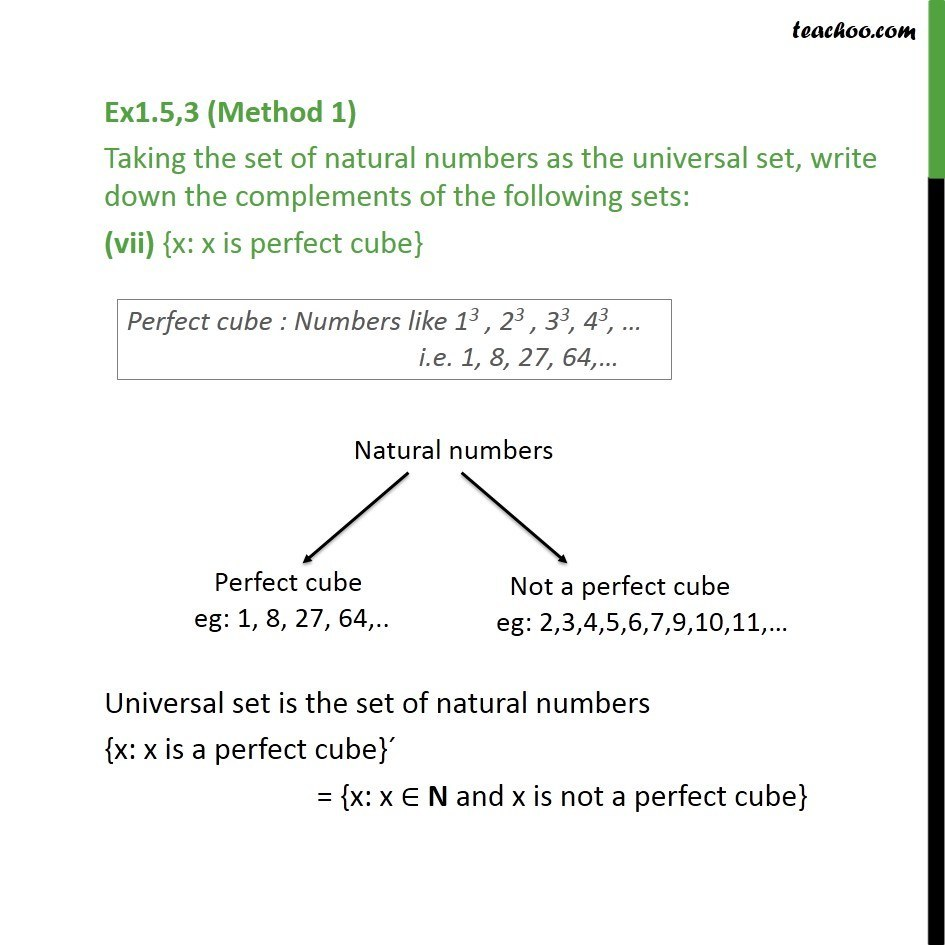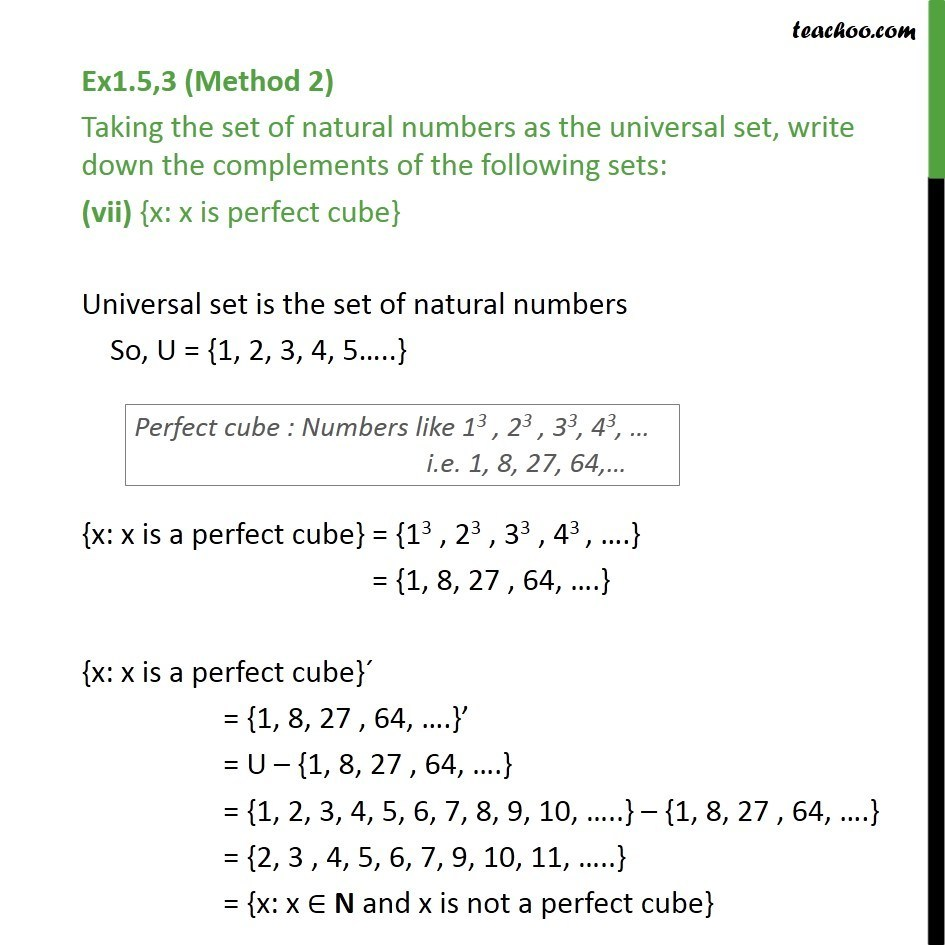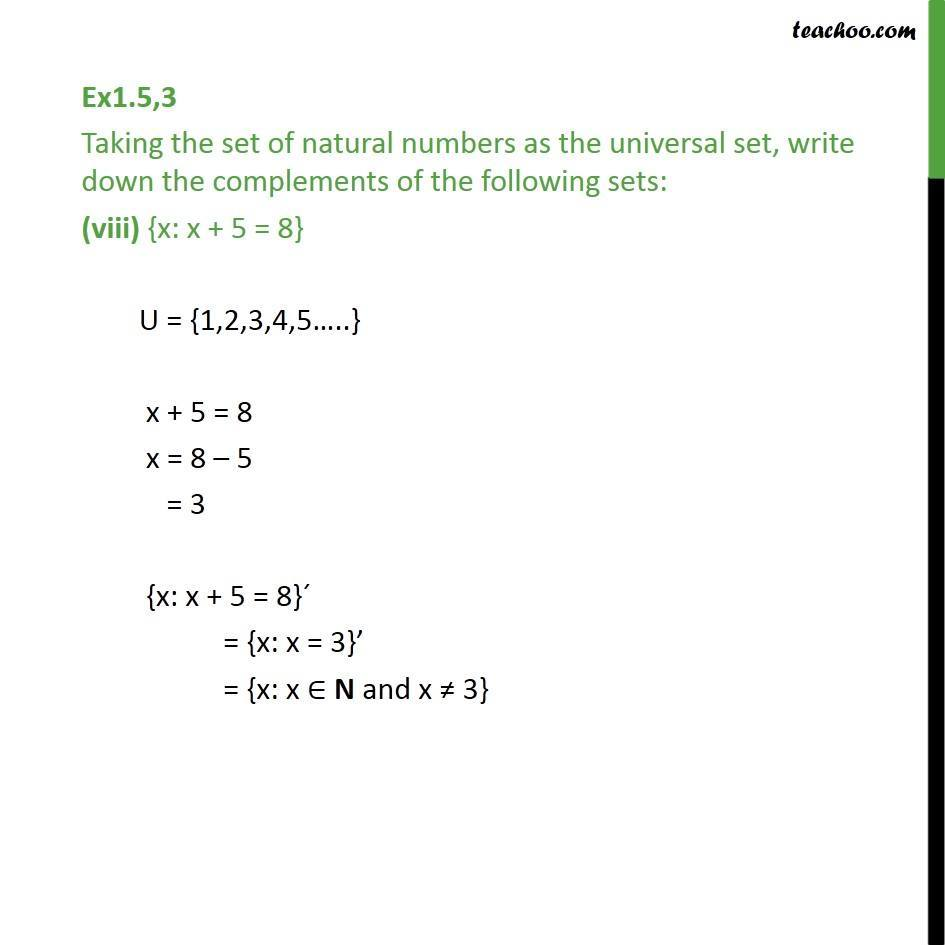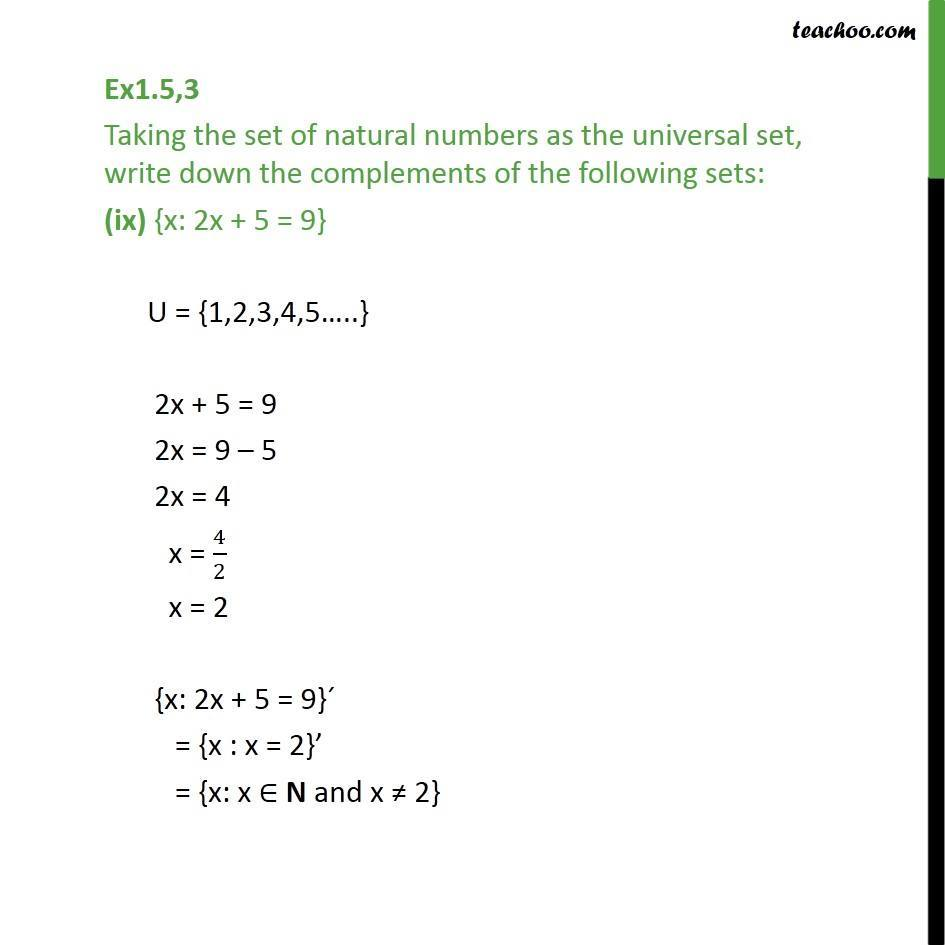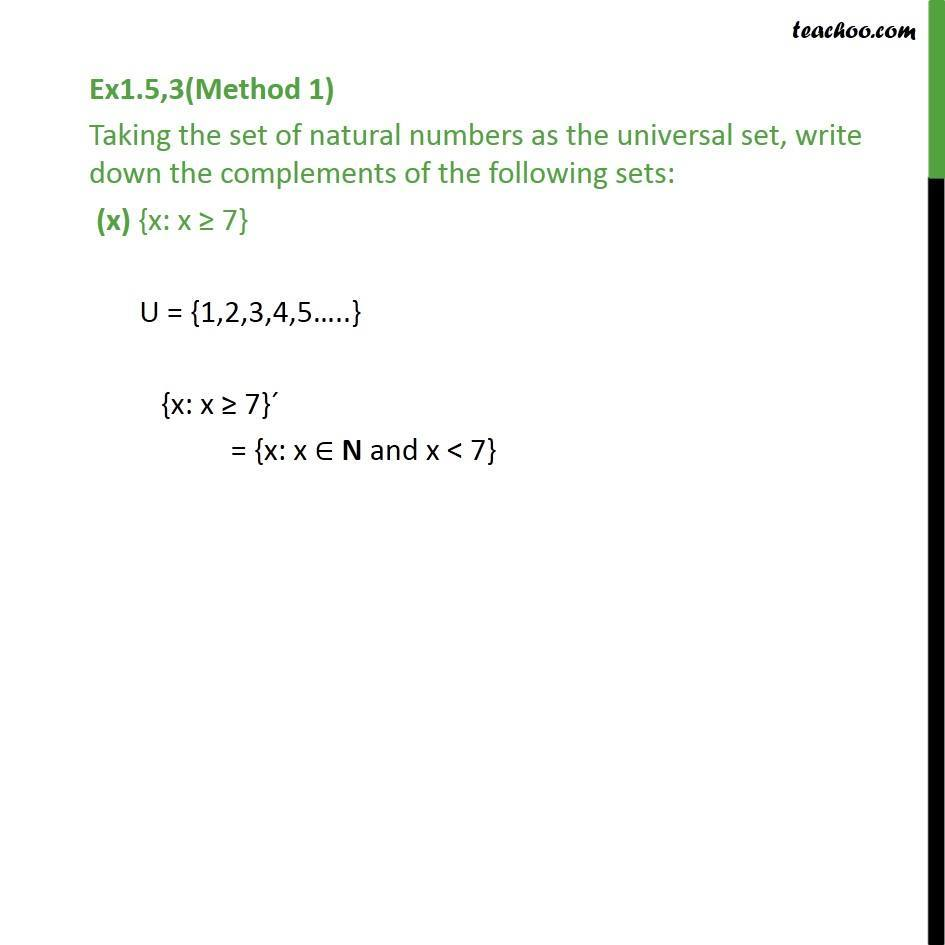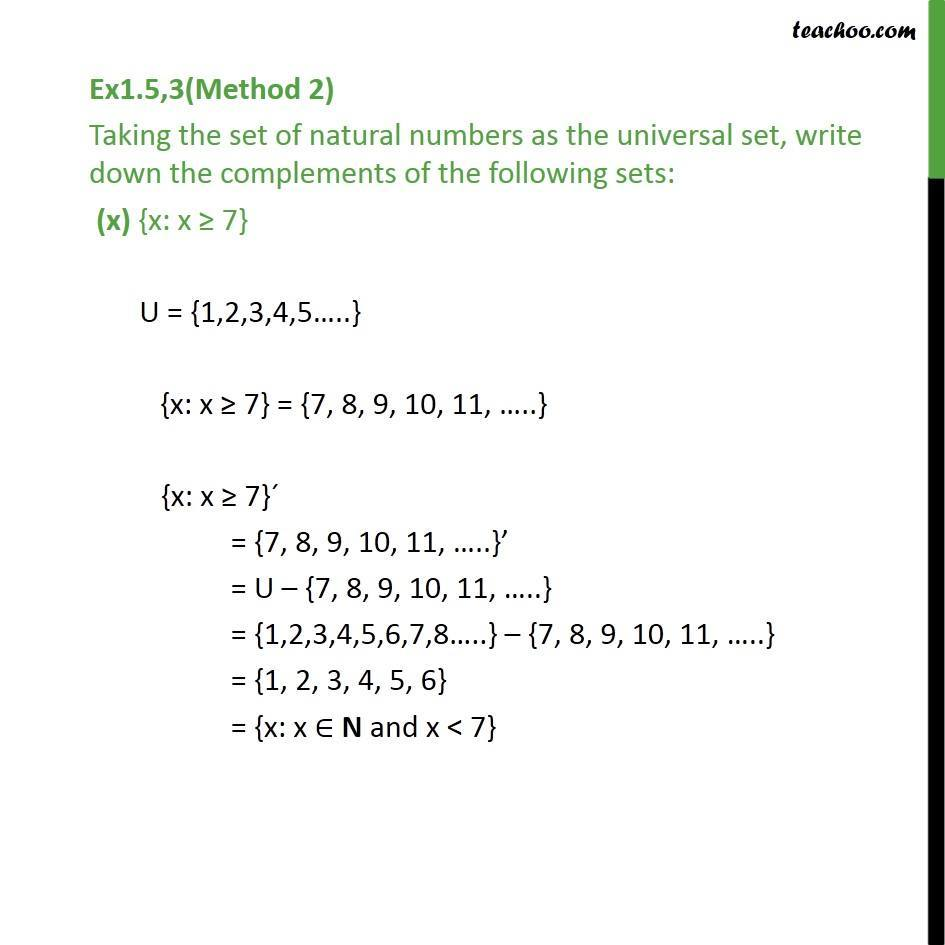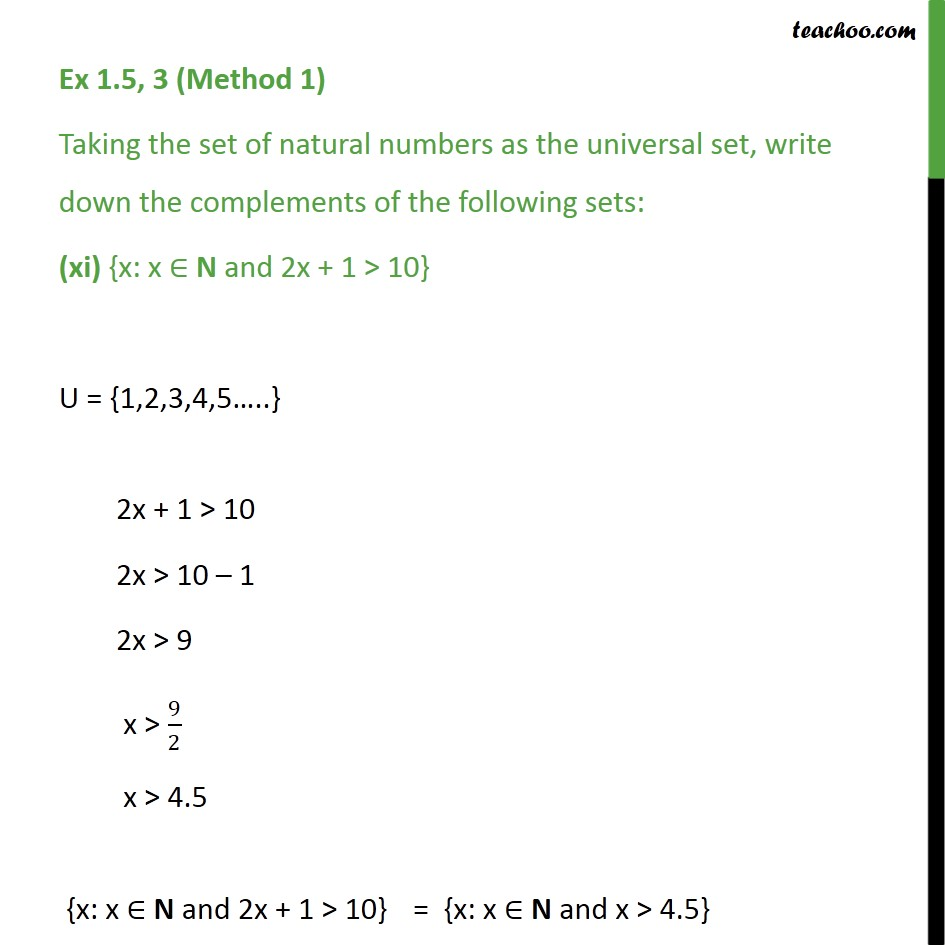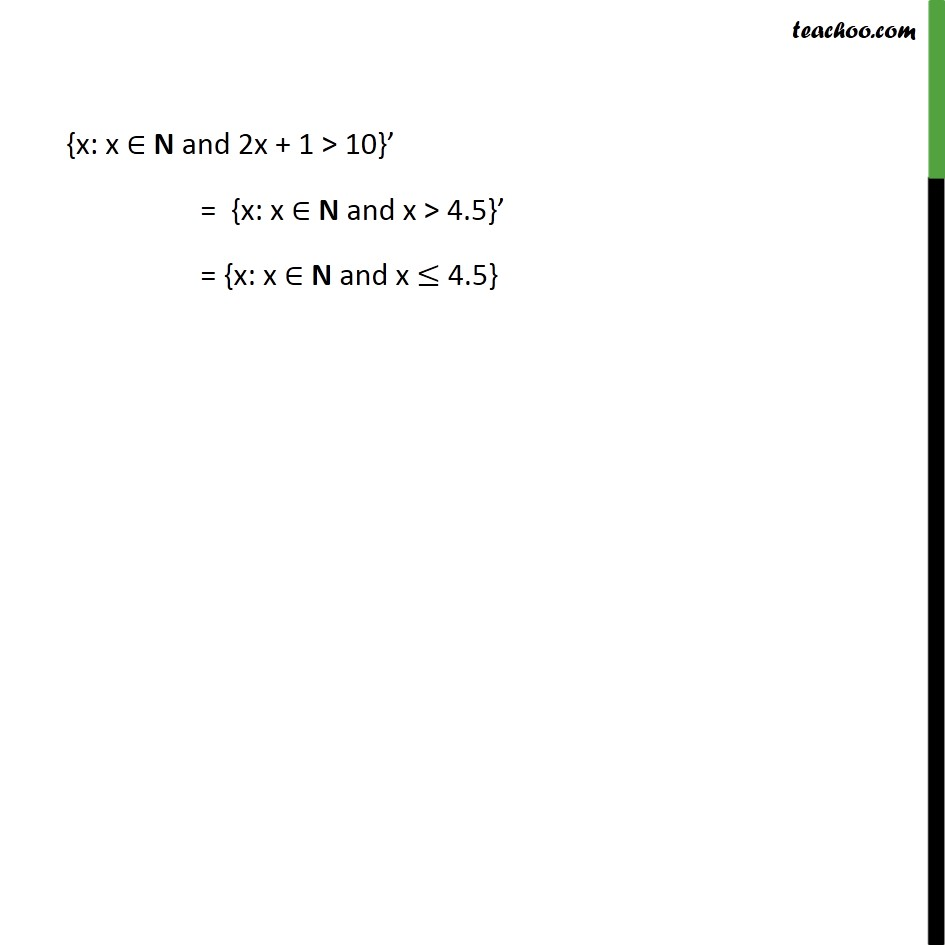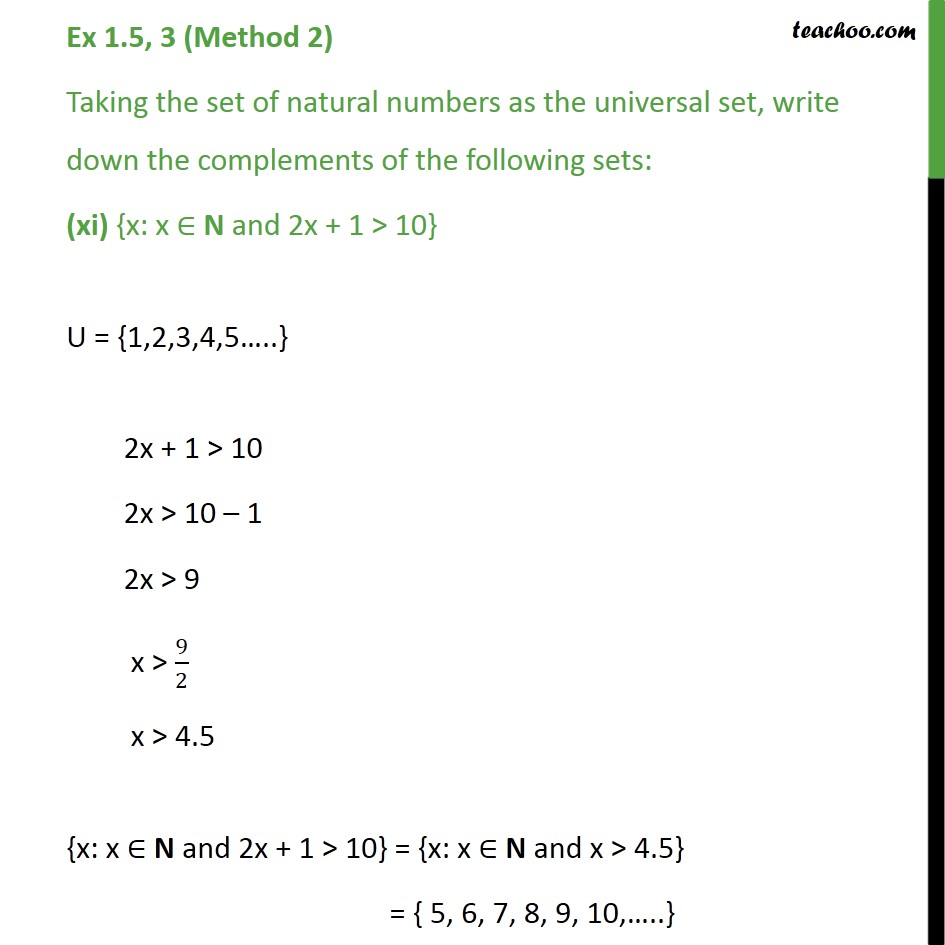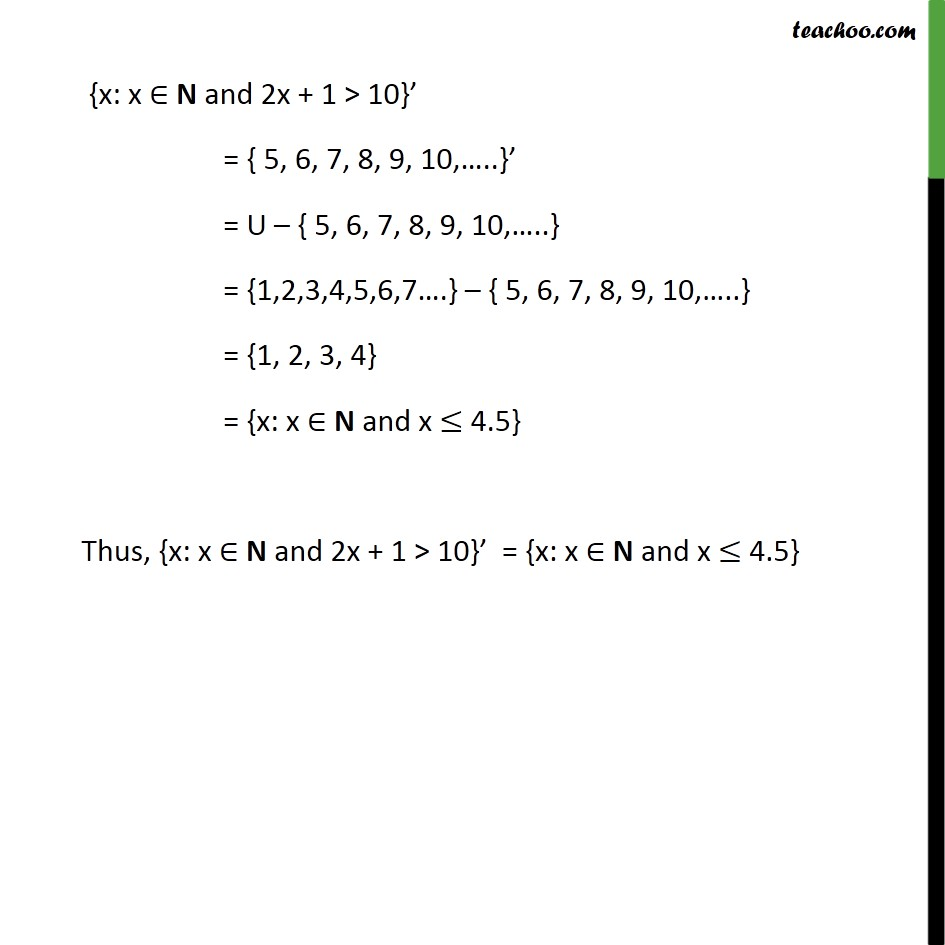1. Chapter 1 Class 11 Sets
2. Concept wise
3. Complement of set

Transcript

Ex1.5,3 (Method 1) Taking the set of natural numbers as the universal set, write down the complements of the following sets: (i) {x: x is an even natural number} Universal set is the set of natural numbers {x: x is an even natural number}’ = {x: x is an odd natural number} Ex1.5,3(Method 2) Taking the set of natural numbers as the universal set, write down the complements of the following sets: (i) {x: x is an even natural number} Universal set is the set of natural numbers So, U = {1, 2, 3, 4, 5…..} {x: x is an even natural number} = {2, 4, 6, 8, 10,….} {x: x is an even natural number}’ = {2, 4, 6, 8, 10,….}’ = U – {2, 4, 6, 8, 10,….} = {1, 2, 3, 4, 5, 6, 7, 8, 9, 10,….} – {2, 4, 6, 8, 10,…} = {1, 3, 5, 7, 9, 11, 13, …..} = {x: x is an odd natural number} Ex1.5,3 (Method 1) Taking the set of natural numbers as the universal set, write down the complements of the following sets: (ii) {x: x is an odd natural number} Universal set is the set of natural numbers {x: x is an odd natural number}’ = {x: x is an even natural number} Ex1.5,3 (Method 2) Taking the set of natural numbers as the universal set, write down the complements of the following sets: (ii) {x: x is an odd natural number} Universal set is the set of natural numbers So, U = {1, 2, 3, 4, 5…..} {x: x is an odd natural number} = {1, 3, 5, 7, 9, 11, 13, …..} {x: x is an odd natural number}’ = {1, 3, 5, 7, 9, 11, 13, …..}’ = U – {1, 3, 5, 7, 9, 11, 13, …..} = {1, 2, 3, 4, 5, 6, 7, 8, 9, 10,….} – {1, 3, 5, 7, 9, 11, 13, …..} = {2, 4, 6, 8, 10,….} = {x: x is an even natural number} Ex 1.5,3(Method 1) Taking the set of natural numbers as the universal set, write down the complements of the following sets: (iii) {x: x is a positive multiple of 3} U = {1,2,3,4,5…..} {x: x is a positive multiple of 3}´ = {x: x ∈ N and x is not a multiple of 3} Ex 1.5,3(Method 2) Taking the set of natural numbers as the universal set, write down the complements of the following sets: (iii) {x: x is a positive multiple of 3} Universal set is the set of natural numbers So, U = {1, 2, 3, 4, 5…..} {x: x is a positive multiple of 3} = {1 × 3 , 2 × 3 , 3 × 3 , 4 × 3 , ….} = {3 , 6 , 9, 12, ….} {x: x is a positive multiple of 3}´ = {3 , 6 , 9, 12, ….}’ = U – {3 , 6 , 9, 12, ….} = {1, 2, 3, 4, 5, 6, 7, 8, 9, 10, …..} – {3 , 6 , 9, 12, ….} = {1, 2, 4, 5, 7, 10, 11, 13,…..} = {x: x ∈ N and x is not a multiple of 3} Ex1.5,3 (Method 1) Taking the set of natural numbers as the universal set, write down the complements of the following sets: (iv) {x: x is a prime number} U is set of natural numbers {x: x is a prime number}´ = {x: x ∈ N and x is a composite number and x = 1} Ex1.5,3 (Method 2) Taking the set of natural numbers as the universal set, write down the complements of the following sets: (iv) {x: x is a prime number} U = {1,2,3,4,5…..} {x: x is a prime number} = {2,3,5,7,11,13…} {x: x is a prime number}´ = {2,3,5,7,11,13…}’ = U – {2,3,5,7,11,13…} = {1, 2, 3, 4, 5, 6, ….} – {2,3,5,7,11,13…} = {1,4,6,8,9,10,12,14,….} = {x: x ∈ N and x is not a prime number and x = 1} = {x: x ∈ N and x is a composite number and x = 1} Ex1.5,3 Taking the set of natural numbers as the universal set, write down the complements of the following sets: (v) {x: x is a natural number divisible by 3 and 5} U = {1,2,3,4,5…..} {x: x is a natural number divisible by 3 and 5}’ = {x: x is a natural number that is not divisible by 3 or 5} Ex1.5,3(Method 1) Taking the set of natural numbers as the universal set, write down the complements of the following sets: (vi) {x: x is a perfect square} U = {1,2,3,4,5…..} {x: x is a perfect square}´ = {x: x ∈ N and x is not a perfect square} Ex1.5,3(Method 2) Taking the set of natural numbers as the universal set, write down the complements of the following sets: (vi) {x: x is a perfect square} Universal set is the set of natural numbers So, U = {1, 2, 3, 4, 5…..} {x: x is a perfect square} = {12 , 22 , 32 , 42 , ….} = {1, 4, 9 , 16, ….} {x: x is a perfect square}´ = {1, 4, 9 , 16, ….}’ = U – {1, 4, 9 , 16, ….} = {1, 2, 3, 4, 5, 6, 7, 8, 9, 10, …..} – {1, 4, 9 , 16, ….} = {2, 3 , 5, 6, 7, 10, 11, …..} = {x: x ∈ N and x is not a perfect square} Ex1.5,3 (Method 1) Taking the set of natural numbers as the universal set, write down the complements of the following sets: (vii) {x: x is perfect cube} Universal set is the set of natural numbers {x: x is a perfect cube}´ = {x: x ∈ N and x is not a perfect cube} Ex1.5,3 (Method 2) Taking the set of natural numbers as the universal set, write down the complements of the following sets: (vii) {x: x is perfect cube} Universal set is the set of natural numbers So, U = {1, 2, 3, 4, 5…..} {x: x is a perfect cube} = {13 , 23 , 33 , 43 , ….} = {1, 8, 27 , 64, ….} {x: x is a perfect cube}´ = {1, 8, 27 , 64, ….}’ = U – {1, 8, 27 , 64, ….} = {1, 2, 3, 4, 5, 6, 7, 8, 9, 10, …..} – {1, 8, 27 , 64, ….} = {2, 3 , 4, 5, 6, 7, 9, 10, 11, …..} = {x: x ∈ N and x is not a perfect cube} Ex1.5,3 Taking the set of natural numbers as the universal set, write down the complements of the following sets: (viii) {x: x + 5 = 8} U = {1,2,3,4,5…..} x + 5 = 8 x = 8 – 5 = 3 {x: x + 5 = 8}´ = {x: x = 3}’ = {x: x ∈ N and x ≠ 3} Ex1.5,3 Taking the set of natural numbers as the universal set, write down the complements of the following sets: (ix) {x: 2x + 5 = 9} U = {1,2,3,4,5…..} 2x + 5 = 9 2x = 9 – 5 2x = 4 x = ﷐4﷮2﷯ x = 2 {x: 2x + 5 = 9}´ = {x : x = 2}’ = {x: x ∈ N and x ≠ 2} Ex1.5,3(Method 1) Taking the set of natural numbers as the universal set, write down the complements of the following sets: (x) {x: x ≥ 7} U = {1,2,3,4,5…..} {x: x ≥ 7}´ = {x: x ∈ N and x < 7} Ex1.5,3(Method 2) Taking the set of natural numbers as the universal set, write down the complements of the following sets: (x) {x: x ≥ 7} U = {1,2,3,4,5…..} {x: x ≥ 7} = {7, 8, 9, 10, 11, …..} {x: x ≥ 7}´ = {7, 8, 9, 10, 11, …..}’ = U – {7, 8, 9, 10, 11, …..} = {1,2,3,4,5,6,7,8…..} – {7, 8, 9, 10, 11, …..} = {1, 2, 3, 4, 5, 6} = {x: x ∈ N and x < 7} Ex1.5,3(Method 1) Taking the set of natural numbers as the universal set, write down the complements of the following sets: (xi) {x: x ∈ N and 2x + 1 > 10} U = {1,2,3,4,5…..} 2x + 1 > 10 2x > 10 – 1 2x > 9 x > ﷐9﷮2﷯ x > 4.5 {x: x ∈ N and 2x + 1 > 10} = {x: x ∈ N and x > 4.5} {x: x ∈ N and 2x + 1 > 10}’ = {x: x ∈ N and x > 4.5}’ = {x: x ∈ N and x ≤ 4.5} Ex1.5,3(Method 2) Taking the set of natural numbers as the universal set, write down the complements of the following sets: (xi) {x: x ∈ N and 2x + 1 > 10} U = {1,2,3,4,5…..} 2x + 1 > 10 2x > 10 – 1 2x > 9 x > ﷐9﷮2﷯ x > 4.5 {x: x ∈ N and 2x + 1 > 10} = {x: x ∈ N and x > 4.5} = { 5, 6, 7, 8, 9, 10,…..} {x: x ∈ N and 2x + 1 > 10}’ = { 5, 6, 7, 8, 9, 10,…..}’ = U – { 5, 6, 7, 8, 9, 10,…..} = {1,2,3,4,5,6,7….} – { 5, 6, 7, 8, 9, 10,…..} = {1, 2, 3, 4} = {x: x ∈ N and x ≤ 4.5} Thus, {x: x ∈ N and 2x + 1 > 10}’ = {x: x ∈ N and x ≤ 4.5}

Complement of set

About the AuthorDavneet Singh
Davneet Singh is a graduate from Indian Institute of Technology, Kanpur. He has been teaching from the past 9 years. He provides courses for Maths and Science at Teachoo.